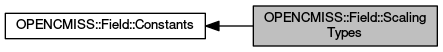OpenCMISS-Iron FORTRAN API Documentation
OPENCMISS::Field::ScalingTypes

Field scaling type parameters. More...

Collaboration diagram for OPENCMISS::Field::ScalingTypes:## Variables

integer(intg), parameter, public opencmiss_iron::cmfe_field_no_scaling = FIELD_NO_SCALING
The field is not scaled. More...

integer(intg), parameter, public opencmiss_iron::cmfe_field_unit_scaling = FIELD_UNIT_SCALING
The field has unit scaling. More...

integer(intg), parameter, public opencmiss_iron::cmfe_field_arc_length_scaling = FIELD_ARC_LENGTH_SCALING
The field has arc length scaling. More...

integer(intg), parameter, public opencmiss_iron::cmfe_field_arithmetic_mean_scaling = FIELD_ARITHMETIC_MEAN_SCALING
The field has arithmetic mean of the arc length scaling. More...

integer(intg), parameter, public opencmiss_iron::cmfe_field_geometric_mean_scaling = FIELD_GEOMETRIC_MEAN_SCALING
The field has geometric mean of the arc length scaling. More...

integer(intg), parameter, public opencmiss_iron::cmfe_field_harmonic_mean_scaling = FIELD_HARMONIC_MEAN_SCALING
The field has geometric mean of the arc length scaling. More...

## Detailed Description

Field scaling type parameters.

OPENCMISS::Field,OPENCMISS

## Variable Documentation

 integer(intg), parameter, public opencmiss_iron::cmfe_field_arc_length_scaling = FIELD_ARC_LENGTH_SCALING

The field has arc length scaling.

OPENCMISS::Field::ScalingTypes,OPENCMISS

Definition at line 3443 of file opencmiss_iron.f90.

 integer(intg), parameter, public opencmiss_iron::cmfe_field_arithmetic_mean_scaling = FIELD_ARITHMETIC_MEAN_SCALING

The field has arithmetic mean of the arc length scaling.

OPENCMISS::Field::ScalingTypes,OPENCMISS

Definition at line 3444 of file opencmiss_iron.f90.

 integer(intg), parameter, public opencmiss_iron::cmfe_field_geometric_mean_scaling = FIELD_GEOMETRIC_MEAN_SCALING

The field has geometric mean of the arc length scaling.

OPENCMISS::Field::ScalingTypes,OPENCMISS

Definition at line 3445 of file opencmiss_iron.f90.

 integer(intg), parameter, public opencmiss_iron::cmfe_field_harmonic_mean_scaling = FIELD_HARMONIC_MEAN_SCALING

The field has geometric mean of the arc length scaling.

OPENCMISS::Field::ScalingTypes,OPENCMISS

Definition at line 3446 of file opencmiss_iron.f90.

 integer(intg), parameter, public opencmiss_iron::cmfe_field_no_scaling = FIELD_NO_SCALING

The field is not scaled.